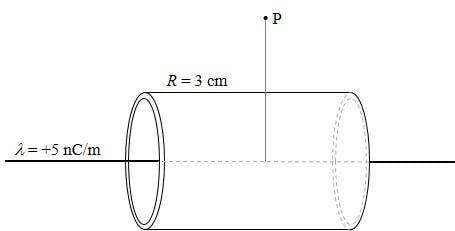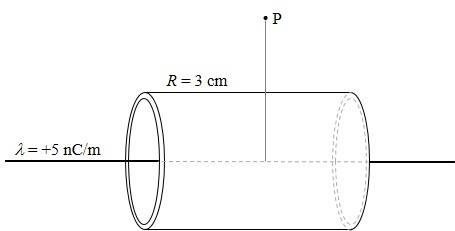# Finding an electric field at a point from a line of charge

• Physiqs

## Homework Statement

A thin insulating rod with charge density lambda=+5nC/m is arranged inside a thin conducting cylindrical shell of radius r =3 cm. The rod and the shell are on the same axis, and infinite in length.
What is electric field at point p? 6cm from the central axis.## Homework Equations

Gauss's law : E*dA = Qenc/epsilon

## The Attempt at a Solution

The question before this was actually to find the sigma.. which was 26.526nC/m^2. So I used sigma in the gauss's law. E*dA( area of cylindrical wall) = (sigma*A)/epsilon and got 2998. However, the answer was exactly the half of that(1499) ... Why?

Just revise the content on field near a non conducting sheet and a conducting sheet. A factor of 2 comes there as there is no field on one side of a conducting sheet as field inside conductor has to be zero. Also i think the answer should be same that you would get in the absence of the infinite cylindrical shell.

## Homework Statement

A thin insulating rod with charge density lambda=+5nC/m is arranged inside a thin conducting cylindrical shell of radius r =3 cm. The rod and the shell are on the same axis, and infinite in length.
What is electric field at point p? 6cm from the central axis.## Homework Equations

Gauss's law : E*dA = Qenc/epsilon

## The Attempt at a Solution

The question before this was actually to find the sigma.. which was 26.526nC/m^2. So I used sigma in the gauss's law. E*dA( area of cylindrical wall) = (sigma*A)/epsilon and got 2998. However, the answer was exactly the half of that(1499) ... Why?

i think you are making an error in calculation...
the line of charge has given charge per unit length. and the Gaussian cylindrical surface (hypothetical) to calculate the flux through it is to be constructed at 6 cm radius so take the area of this surface times the electrical intensity and apply Gauss theorem.

very close to a non-conducting sheet of charge E = σ/ε₀ and for a conducting sheet of charge E' = σ/(2ε₀)

Last post was not correct. It may be read as:

very close to a non-conducting sheet of charge E = σ/(2ε₀) and for a conducting sheet of charge E' = σ/ε₀